# Moon

We see Moon in the perspective angle 28'. Moon's radius is 1740 km at the time of the full moon. Calculate the mean distance of the Moon from the Earth.

x =  425520 km

### Step-by-step explanation: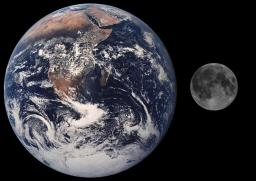Did you find an error or inaccuracy? Feel free to write us. Thank you!Tips to related online calculators
Do you want to convert length units?

#### You need to know the following knowledge to solve this word math problem:

We encourage you to watch this tutorial video on this math problem:

## Related math problems and questions:

• The spacecraft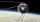The spacecraft spotted a radar device at altitude angle alpha = 34 degrees 37 minutes and had a distance of u = 615km from Earth's observation point. Calculate the distance d of the spacecraft from Earth at the moment of observation. Earth is considered a
• What percentageWhat percentage of the Earth’s surface is seen by an astronaut from a height of h = 350 km. Take the Earth as a sphere with the radius R = 6370 km
• Rotation of the EarthCalculate the circumferential speed of the Earth's surface at a latitude of 61°​​. Consider a globe with a radius of 6378 km.
• TreeBetween points A and B is 50m. From A we see a tree at an angle 18°. From point B we see the tree in three times bigger angle. How tall is a tree?
• Right angleIn a right triangle ABC with a right angle at the apex C, we know the side length AB = 24 cm and the angle at the vertex B = 71°. Calculate the length of the legs of the triangle.
• Felix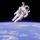Calculate how much land saw Felix Baumgartner after jump from 32 km above ground. The radius of the Earth is R = 6378 km.
• Earth parallelEarth's radius is 6370 km long. Calculate the length parallel of latitude 50°.Calculate the content of a regular 15-sides polygon inscribed in a circle with radius r = 4. Express the result to two decimal places.
• FighterA military fighter flies at an altitude of 10 km. The ground position was aimed at an altitude angle of 23° and 12 seconds later at an altitude angle of 27°. Calculate the speed of the fighter in km/h.
• SteepleWe see the church tower from the road at an angle of 52°. When we zoom out to 29 meters away, it can be seen at an angle of 21°. How high is it?
• Right angled triangle 3Side b = 1.5, hypotenuse angle A = 70 degrees, Angle B = 20 degrees. Find its unknown sides length.
• Elevation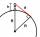What must be the elevation of an observer in order that he may be able to see an object on the earth 536 km away? Assume the earth to be a smooth sphere with radius 6378.1 km.
• Space diagonal anglesCalculate the angle between the body diagonal and the side edge c of the block with dimensions: a = 28cm, b = 45cm and c = 73cm. Then, find the angle between the body diagonal and the plane of the base ABCD.
• Length of the chord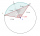Calculate the length of the chord in a circle with a radius of 25 cm with a central angle of 26°.
• RectangleCalculate the length of the side GN and diagonal QN of rectangle QGNH when given: |HN| = 25 cm and angle ∠ QGH = 28 degrees.
• CloudsApproximately at what height is the cloud we see under an angle of 26°10' and see the Sun at an angle of 29°15' and the shade of the cloud is 92 meters away from us?
• Earth's circumference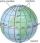Calculate the Earth's circumference of the parallel 48 degrees and 10 minutes.